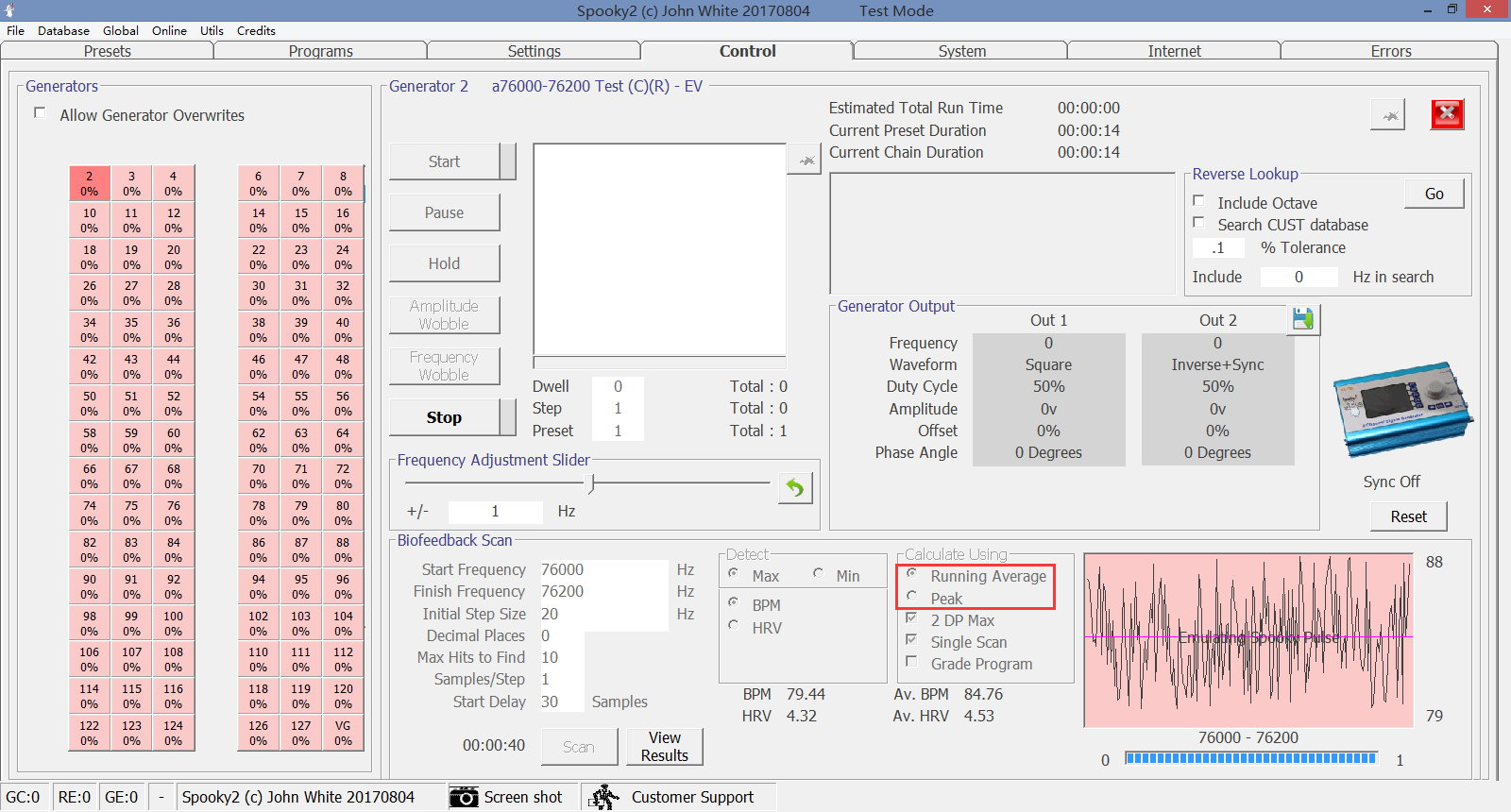# How Is the Hits Value of Biofeedback Scans Calculate 2

The biofeedback has two methods of analysis, each with two methods of calculation.

Beats per minute – peak, which will return #s that show the heart rate at the time of the hit.

Beats per minute – running average, which will return the deviation from the running average and will be lower numbers like you are seeing.

For instance, if my average heart rate is 60, and a hit is measured with a rate that spiked to 72, then the hit will be returned either as:
72 for BPM – peak
12 for BPM – running average

Heart Rate Variance – peak, which returns small numbers since this is the variance from what is measured prior.

For instance if my heart rate was 60, and then the next frequency is measured at 68, the HRV is 8.

Heart Rate Variance – running average, which will return even smaller numbers as this is the variance from the running average.

If my heart rate was 60, but my running average is 65, and the next frequency is measured at 68, the HRV will be 3.Authored by: Jeff Kaczor
Source: http://www.spooky2.com/forums/viewtopic.php?f=39&t=7408

1.1.